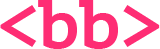backbencher.dev

# Number Data Type

In most of the programming languages, integer values and floating-point(numbers with decimal) values are treated differently. The amount of memory used for both types also varies. But in JavaScript, there is no distinction between integer values and floating-point values.

## Memory and Range

In JavaScript, both integer and floating-point values are treated in the same way. Therefore, considering the worst case, the memory allocation for a number type should consider floating-point values. Each number in JavaScript occupies 64 bit of memory. The numbers are stored in Double-precision floating-point format as per IEEE 754 standard.

In case of integers, JavaScript can accurately represent all integers between -9007199254740992(-253) and 9007199254740992(253), inclusive. Beyond this range, the precision might lose. Let us try adding one to the maximum range.

``console.log(9007199254740992+1); // 9007199254740992``

The expected output is 9007199254740993. But the actual output is 9007199254740992.

## Numeric literal

When a number appears directly in a program, it is called a numeric literal. JavaScript supports numeric literals in several formats.

``````12      // Base 10 integer literal
0xFF    // Base 16 integer literal
0377    // Base 8 integer literal
3.14    // Floating-Point literal
6.73e5  // Floating-Point literal``````

### Integer literal

Integers in JavaScript can be represented in 3 ways.

• Decimal
• Octal

Decimal numbers are sequence of digits with base 10. When we say integers, what come to our minds is decimal numbers. Examples:

``````0
23
-46
100000``````

Hexadecimal integers have their base as 16. Hexadecimal integers can have digits from 0 to 9 and alphabets A to F. In JavaScript, we denote hexadecimal integers by placing either `0x` or `0X` before the number.

``````console.log(0xFF);  // 255
console.log(0XA);   // 10``````

Even though we log a hexadecimal integer, `log` method converts it to a decimal before printing in console.

Some JavaScript engines support Octal format. Octal numbers have there base as 8. A numeric literal is marked as octal by prepending a zero(0).

``console.log(077); // 63``

In Google Chrome, above line prints `63`. It is supported by Chrome's V8 JavaScript engine. Now prepending a zero to mark a number as octal can lead to unexpected errors. Therefore, octal specification is not there in ECMAScript specification. It is not advised to use octal format because of non uniform support across JavaScript engines.

If we use an octal number notation with a non-octal digit, JavaScript engine does not throw an error. Instead, it simply treats the number as a decimal.

``````console.log(077);   // 63
console.log(099);   // 99``````

### Floating-point Literal

Floating-point literals are real numbers that contain a decimal point. They contain an integer part, a decimal point and a fractional part. Some examples:

``````2.78
.912``````

Floating-point literals can also be represented using exponential notation. Exponential notation is an `e`(or `E`) followed by a positive or negative integer. Here is an example:

``````3.67e5  // 367000
3.67e-5 // .0000367``````

`e5` denotes multiply the number `3.67` with 100000. In the same line, `e-5` denotes divide the number `3.67` by 100000.

## NaN

`NaN` is a predefined global variable which holds a Not a Number value. Sometimes, JavaScript need to tell user that the evaluated result is not a number. NaN is for that purpose.

``````console.log("hello" * 2); // NaN
console.log(0/0);         // NaN``````

So is `NaN` a variable or value? It is both a variable and value. It is something like, there is a variable `a` which holds the value `"a"`. Coming back to NaN, NaN is a global variable. So we can find it as a property of `window` object. The value that variable holds is `NaN` which is a representation for Not a Number in JavaScript.

``console.log(window.NaN); // NaN``

There is also a property for `Number` object called `NaN`. Again, the value of that property is `NaN`.

``console.log(Number.NaN); // NaN``

### NaN == NaN

So does NaN evaluates to `true` when compared with itself? No. That makes sense because NaN is just a representation of Not a Number. For example, `"a"` is not a number. `"b"` is not a number. So does that mean that `"a"` and `"b"` are equal? No. Just like that, NaN is not equal to NaN.

``console.log(NaN == NaN); // false``

`NaN` does not compare true with any value, including itself. So we cannot check if a value is `NaN` by comparing it with `NaN`. Instead we can compare a value with itself, and if it returns `false`, then that value is definitely `NaN`.

``````var x = NaN;
console.log(x != x); // true``````

We can also find if a value is `NaN` by using `isNaN()` method. `isNaN()` method returns `true` if the argument passed to the method is `NaN` or a value which cannot be converted to a number.

``````var x = NaN;
console.log(isNaN(x));        // true
console.log(isNaN("apple"));  // true
console.log(isNaN("123"));    // false. It can be converted to 123
console.log(isNaN(true));     // false. true can be converted to 1
console.log(isNaN({}));       // true``````

### NaN as Variable

As we discussed in earlier section, `NaN` is a predefined global variable. In ECMAScript 3, we could both read/write that predefined global variable. But in ECMAScript 5, `window.NaN` is read only. It is not possible to edit the value of `window.NaN`.

``````NaN = 46;
console.log(NaN); // NaN``````

## Type conversion to Number data type

There are different techniques to convert a non-number data type to number data type.

### Multiply with one

`*` is an operator in JavaScript to do multiplication. We know that a number multiplied with one returns the same number. Also, since multiplication(`*`) applies only to numbers, JavaScript automatically converts all operands of `*` operator to number data type. We use this automatic type conversion feature in JavaScript to convert any data type to a number type.

``````// Boolean to Number
console.log(true * 1);  // 1
console.log(false * 1); // 0

// String to Number
console.log("123" * 1); // 123
console.log("abc" * 1); // NaN

// Null to Number
console.log(null * 1);  // 0

// Undefined to Number
console.log(undefined * 1); // NaN``````